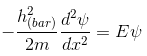# Problem: Schroedinger equation:Express the energy of the particle E in terms of the wavenumber of the particle k.Express your answer in terms of wave number k, mass m, and Planck's constant divided by 2pi:h(barr).

###### FREE Expert Solution

Wavenumber:

$\overline{){\mathbf{k}}{\mathbf{=}}\frac{\mathbf{2}\mathbf{\pi }}{\mathbf{\lambda }}}$

Momentum:

$\overline{){\mathbf{p}}{\mathbf{=}}\frac{\mathbf{h}}{\mathbf{\lambda }}}$

$\begin{array}{rcl}\mathbf{E}& \mathbf{=}& \frac{{\mathbf{p}}^{\mathbf{2}}}{\mathbf{2}\mathbf{m}}\end{array}$

86% (197 ratings)###### Problem Details

Schroedinger equation:Express the energy of the particle E in terms of the wavenumber of the particle k.

Express your answer in terms of wave number k, mass m, and Planck's constant divided by 2pi:h(barr).